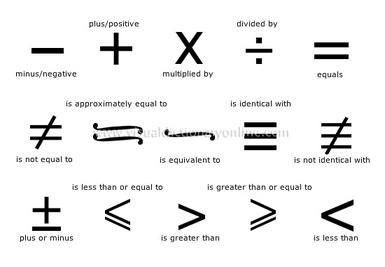Math Signs in English

Math Signs in English

minus/negative, plus/positive, multiplied by, divided by, equals, is not equal to, is appoximately equal to, is less than, is greater than or equal, is greater than, plus or minus and etc. Follow the image for much more and visual expression;